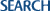> > Archive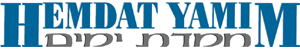# Shabbat Parashat Korach | 5769

## Hemdat Hadaf Hayomi: Calculation of Ona'ah

Baba Metziah 50-56

This week in the Daf Hayomi, the Gemara deals primarily with the prohibition of ona'ah.  Ona'ah is a prohibition against buying or selling an object not at its market price. The Gemara (61a) understands ona'ah to be a form of theft. The reason for this is that a sale is considered a deal in which the buyer gives the seller the value of the object he is selling. Thus, if the buyer receives a price higher than the market price of the object, he is stealing from the buyer the difference, because he didn't give the buyer an object of matching value. Likewise, if the buyer paid less then the price of object, he is stealing that part of the value of the object for which he did not pay.

What happens if an object was not sold at its market price? The conclusion of the Gemara (50b) is as follows: If the difference between the market price of the object and the sale price is less than a sixth, then the sale stands, since people forgive a small difference. If the difference is exactly a sixth, then the sale stands, but the difference must be refunded or paid. If the difference is more then a sixth, then the sale is cancelled.

How is a sixth calculated? The Gemara (49b) quotes an argument regarding this between Rav and Shmuel. According to Rav, a sixth is calculated as a sixth of the market price of the object. For example, if the market price is \$60, then a sixth is \$10. If it was sold at \$70, then the seller has to return \$10 to the buyer, and if it was sold at \$50 the buyer has to pay another \$10 to the seller. If it was sold for more then \$50 or less then \$70 the sale stands as is. If it was sold at less then \$50 or more the \$70, the sale is cancelled.

Shmuel agrees with the calculation of Rav, but adds that there is another form of calculation. According to his opinion, one can calculate a sixth also as a sixth of the sale price. Thus, if an object was sold for \$60 and its market price was \$50, this too is considered a difference of a sixth and the seller must return \$10 to the buyer. Likewise, if an object was sold for \$60, and its market price was \$70, this is also considered a difference of a sixth, and the buyer must pay an additional \$10 to the seller. The Halacha is ruled in accordance with the opinion of Shmuel (Choshen Mishpat 227, 2).

The problem with the opinion of Shmuel is in a case where, according to both calculations, there isn't exactly a sixth, and the two calculations give contradicting results. For example, if an object whose market price is \$60 is sold for \$51, the difference is \$9. If we calculate a sixth as a sixth of the market price (60/6=10), then the difference is less then a sixth, meaning the sale should stand as is. However, if we calculate a sixth as a sixth of the price it was sold at (51/6=8.5), then the difference is more then a sixth meaning the sale should be cancelled.

There are two opinions as to how to resolve this problem. According to the Magid Mishne (Mechira 12, 3), the entire range between the two calculations of a sixth is considered as a difference of a sixth, in which case the sale stands but the difference must be paid, Thus, in our example, even though the difference is not exactly a sixth according to either calculation, since it falls in between the two calculations, it is considered a difference of a sixth, and the buyer will have to pay an additional \$9 to the seller.

According to the Mordechai (Baba Metzia 304, and this appears to be the opinion of the Rambam Mechira 12, 3), the primary calculation is a sixth of the market price, and we only calculate based on the sale price in a case where the difference would be exactly a sixth, according to that calculation. In all other cases, the calculation is done as a sixth of the market price of the object. Thus, in our example, since the difference (\$9) is less then a sixth of the market price of the object (\$10), the sale stands as is. The Ramma (ibid, 4) rules in accordance with the Mordechai.Top of page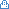Print this page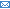Send to friend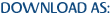###This edition of Hemdat Yamim is dedicated to the memory of

R ' Meir ben Yechezkel Shraga  Brachfeld

o.b.m

Hemdat Yamim is endowed by
Les & Ethel Sutker of Chicago, Illinois in loving memory of
Max and Mary Sutker

and Louis and Lillian Klein, z”l.

site by entry.